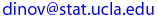Ivo Dinov
UCLA Statistics, Neurology, LONI
 Courses SOCR Ivo Dinov's Home SiteMap Software Contact

Statistics 13
Sec. 02
Fall 2005
##### Introduction to Statistical Methods for the Life and Health Sciences

Text  & Syllabus

Instructor: Ivo Dinov

Textbook:
Statistics for the Life Sciences, 3/E
Myra L. Samuels, (Emerita)
Jeffrey A. Witmer, Oberlin College

Tentative schedule of topics to be covered

1. Introduction. Statistics and the Life Sciences. Examples and Overview.

2. Description of Populations and Samples. Introduction. Frequency Distributions: Techniques for Data. Frequency Distributions: Shapes and Examples. Descriptive Statistics: Measures of Center. Boxplots. Measures of Dispersion. Effect of Transformation of Variables (Optional). Samples and Populations: Statistical Inference.

3. Random Sampling, Probability, and the Binomial Distribution. Probability and the Life Sciences. Random Sampling. Introduction to Probability. Probability Trees. Probability Rules (Optional). Density Curves. Random Variables. The Binomial Distribution. Fitting a Binomial Distribution to Data (Optional).

4. The Normal Distribution. The Normal Curves. Areas Under a Normal Curve. Assessing Normality. The Continuity Correction (Optional).

5. Sampling Distributions. Basic Ideas. Dichotomous Observations. Quantitative Observations. Illustration of the Central Limit Theorem (Optional). The Normal Approximation to the Binomial Distribution (Optional).

6. Confidence Intervals. Statistical Estimation. Standard Error of the Mean. Confidence Interval. Planning a Study to Estimate. Conditions for Validity of Estimation Methods. Confidence Interval for a Population Proportion.

7. Comparison of Two Independent Samples. Standard Error of (y1 - y2). Confidence Interval. Hypothesis Testing: The t-test. Further Discussion of the t-test. One-Tailed Tests. More on Interpretation of Statistical Significance. Planning for Adequate Power (Optional). Student's t: Conditions and Summary. More on Principles of Testing Hypotheses. The Wilcoxon-Mann-Whitney Test.

8. Statistical Principles of Design. Observational Studies. Experiments. Restricted Randomization: Blocking and Stratification. Levels of Replication. Sampling Concerns (Optional).

9. Comparison of Paired Samples. The Paired-Sample t-Test and Confidence Interval. The Paired Design. The Sign Test. The Wilcoxon Signed-Rank Test. Further Considerations in Paired Experiments.

10. Analysis of Categorical Data. Inference for Proportions: The Chi-Squared Goodness-of-Fit Test. The Chi-Squared Test for a 2 X 2 Contingency Table. Independence and Association in a 2 X 2 Contingency Table. Fisher's Exact Test (Optional). The r x k Contingency Table. Applicability of Methods. Confidence Interval for a Difference Between Proportions. Paired Data and 2 X 2 Tables (Optional). Relative Risk and the Odds Ratio (Optional). Summary of Chi-Squared Tests.

11. Comparing the Means of Many Independent Samples. The Basic Analysis of Variance. The Analysis of Variance Model (Optional). The Global F-Test. Applicability of Methods. Two-Way ANOVA (Optional). Linear Combinations of Means.

12. Linear Regression and Correlation. The Fitted Regression Line. Parametric Interpretation of Regression: The Linear Model. Statistical Inference Concerning B1. The Correlation Coefficient. Guidelines for Interpreting Regression and Correlation. Perspective. Summary of Formulas.

13. A Summary of Inference Methods. Data Analysis Samples.

 Ivo Dinov's Home http://www.stat.ucla.edu/~dinov Visitor number, since Jan. 01, 2002 © I.D.Dinov 1997-2006
Last modified on by